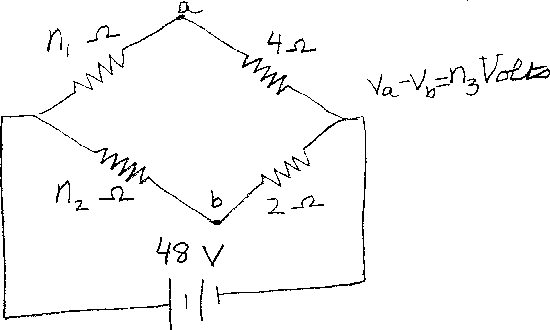Problem B8: Consider the circuit shown above that has 4 resistors connected to a 48 Volt battery. The resistor in the upper left has a resistance of n1 Ohms. The resistor in the lower left has a resistance of n2 Ohms. What is the Voltage difference between point a and point b in the circuit? If Va-Vb=n3 Volts, what is n3?n1 = n2 = Input n3:
If you are currently in my class, you can record your grade by entering your name and student ID number (without the leading zeros) below and clicking on "record grade".
 First Name = Last Name = ID = Problem: